# Test: Basic Electronics- 2

## 20 Questions MCQ Test Mock Test Series for SSC JE Electrical Engineering | Test: Basic Electronics- 2

Description
Attempt Test: Basic Electronics- 2 | 20 questions in 12 minutes | Mock test for SSC preparation | Free important questions MCQ to study Mock Test Series for SSC JE Electrical Engineering for SSC Exam | Download free PDF with solutions
QUESTION: 1

### At much lower temperature, semiconductors behave as:

Solution:

At lower temperatures, the electrons in valence band do not have sufficient energy to jump into conduction band. Thus carrier concentration decreases at lower temperatures and semiconductors behaves as insulators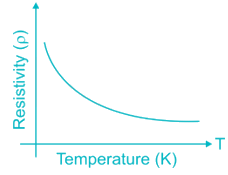QUESTION: 2

### In the silicon crystal structure, the recombination rate is proportional to the number of;

Solution:

In an intrinsic semiconductor (un doped silicon) the number of electrons and the number of holes are equal. Hence recombination rate depends on both the number of electrons & number of holes.

In case of doped semiconductors, the recombination rate of the majority carriers are dependent on minority carrier density.

QUESTION: 3

### The Fermi level EF in an intrinsic semiconductor, if effective masses of holes and electrons are same is:

Solution:

The fermi level (EF) in an intrinsic semiconductor is given by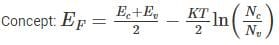Where Ec = Energy of conduction band minima

Ev = Energy of valence band maxima

K = Boltzman constant

T = Temperature

Nc = density of states in conduction band

Nv = density of states in valence band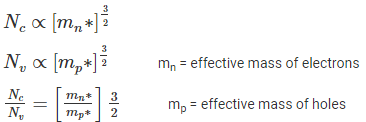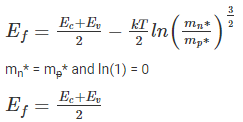QUESTION: 4

Built- in potential Vof a junction depends on:

Solution:

The built in potential V0 of a junction is given by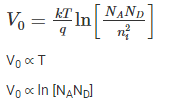So built-in potential depends on both doping densities & temperature

QUESTION: 5

During reverse bias operation of PN junction, a low current flows known as…….. which is……… barrier voltage:

Solution:

The reverse bias characteristics of a p – n junction diode is shown in the figure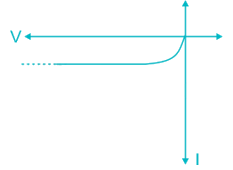From the figure it is clear that the reverse current is independent of voltage.

QUESTION: 6

The depletion region of PN junction is consist of:

Solution: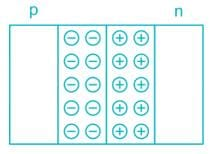The holes from p-region cross the p-n Junction and recombine with electrons on the n-region. Similarly the electrons form n-region cross the junction & recombine with holes in p-region. Leaving immobile ions behind.

Thus the region near the junction is devoid of any mobile charge carriers, due to their recombination. Hence Depletion region of p-n Junction consists of immobile charges.

QUESTION: 7

A centre tapped full wave rectifier output contains only-

Solution: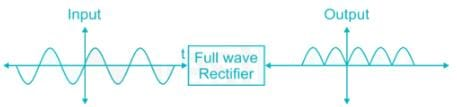Since the output of full wave rectifier is an even function. Hence from Fourier series Analysis we know that even function have even harmonics thus output of FWR contains only even harmonics.

QUESTION: 8

What is the effect on the diode current in a forward biased photo diode with increase in incident light intensity?

Solution:

When the photodiode is forward biased it acts as a normal diode and the incident light intensity does not affect the diode current

QUESTION: 9

Which diode has negative resistance region in its characteristics?

Solution:

Tunnel diode characteristics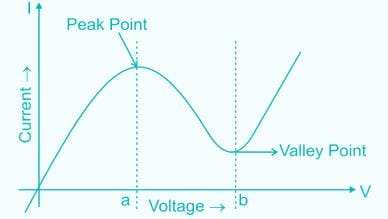In Region between Peak Point & valley Point the current in Tunnel diode decrease with increase in forward Bias voltage. Thus Tunnel diode shows -ve resistance between peak point & valley point

QUESTION: 10

The ripple factor of a power supply is a measure of

Solution: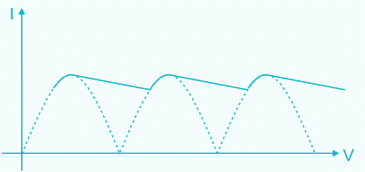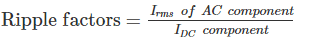Thus if the ripple factor is less, the power supply has less AC components and power supply output is more pure (i.e more DC without much fluctuations)

Thus ripple factor is indication of purity of output of power supply

QUESTION: 11

Which of the following diode is used for voltage stabilization?

Solution:

The I - V characteristic of Zener diode is shown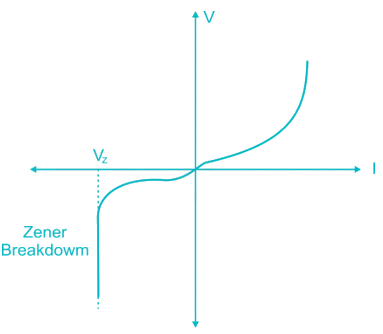When Zener diode is reversed Biased the voltage across it is constant (V2).

Hence Zener Diode on reverse Biasing acts as voltage Stabilizer

QUESTION: 12

Of the three BJT configurations CB, CE and CC:

Solution: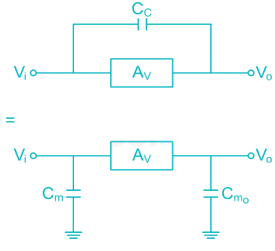Av = gain of Amplifier

Miller Capacitance

CM = CC (1 + AV)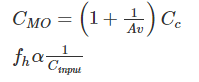The increase in input capacitance decreases the upper cut off frequency

In case of CB configuration there is no miller capacitance between input and output & hence upper cut off frequency (fH) is high & higher Bandwidth

QUESTION: 13

A transistor connected in common base configuration has:

Solution: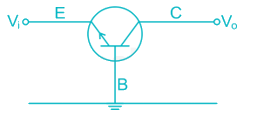Input Terminal Emitter – Base (EB)

Output Terminal Collector – Base (CB)

Since for Amplification Application in BJT

EB Junction → Forward Biased (Low Impedance)

CB Junction → Reversed Biased (High Impedance)

Input Impedance is low

Output Impedance is high

QUESTION: 14

Which of the following is not possible BJT configuration?

Solution:

The 3 configurations in BJT are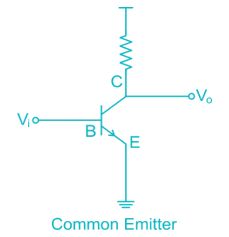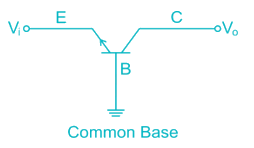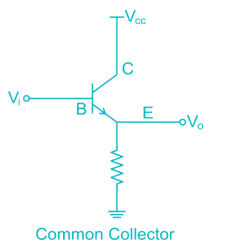The term “Common” refers to the Common terminal through which input is given and from which output is withdrawn i.e. common terminal between input and output.

The term common current is logically incorrect and is not a BJT Configuration.

QUESTION: 15

When a BJT is used as switch, its mode of operation switches between:

Solution:

Different modes of BJT operations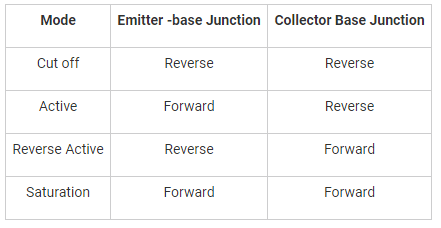For switching Application BJT is operated in cut off (off switch) and saturation (on switch)

QUESTION: 16

For a transistor to act as current amplifier.

Solution: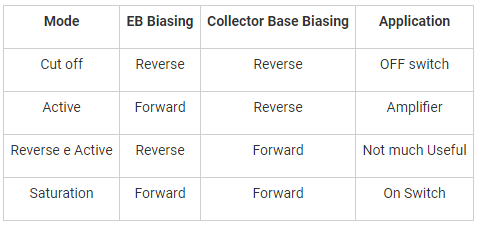For any amplification, (voltage or current), the transistor should be operated in active region ie. emitter base junction is forward biased and collector base junction is reverse biased

QUESTION: 17

The upper cut-off frequency of an RC coupled amplifier mainly depends upon:

Solution:

The Upper cut off frequency of R-C coupled multistage Amplifier is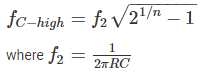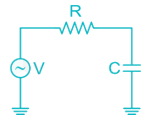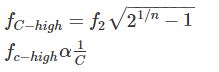Thus upper – cut off frequency depends on coupling capacitors

QUESTION: 18

The cascade amplifier is a multistage configuration of:

Solution: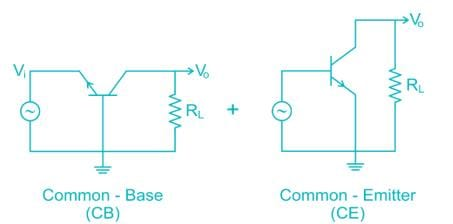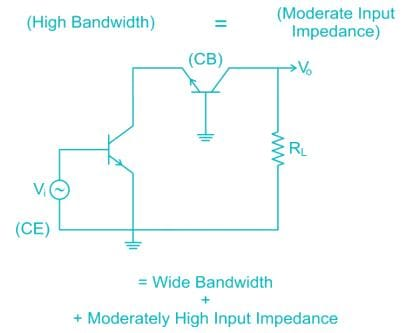Hence cascade is CE – CB Configuration

QUESTION: 19

A Schottky diode is a

Solution:

It is a majority carrier device. It is a semiconductor diode with a very fast switching action, but a low forward voltage drop. When a current flows through the diode there is a small voltage drop across the diode terminals. In a normal diode, the voltage drop is between 0.6 to 1.7 volts, while in a Schottky diode the voltage drop normally ranges between 0.15 and 0.45volts. This lower voltage drop provides higher switching speed and better system efficiency.

In Schottky diode, a semiconductor-metal junction is formed between a semiconductor and a metal, thus creating a Schottky barrier. The N-type semiconductor acts as a cathode and the metal side acts as the anode of the diode. The Schottky barrier diode is a unidirectional device conducting current flows only in one direction.

QUESTION: 20

at room temperature intrinsic carrier concentration is higher in germanium than in silicon because __________.

Solution:

Carrier concentrations depends on the energy gap in the intrinsic semiconductor. In the case of Ge and Si the intrinsic carrier concentration of Ge is higher than that of Si because the energy gap in Ge is smaller than that of Si.Use Code STAYHOME200 and get INR 200 additional OFF Use Coupon Code